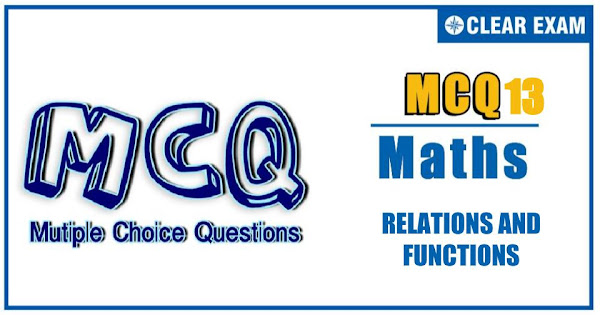## RELATIONS AND FUNCTIONS Quiz-13

In mathematics, a function can be defined as a rule that relates every element in one set, called the domain, to exactly one element in another set, called the range. For example, y = x + 3 and y = x2 – 1 are functions because every x-value produces a different y-value. A relation is any set of ordered-pair numbers..

Q1. The entire graph of the equation y=x^2+kx-x+9 is strictly above the x-axis if and only if
•  k<7
•   -5
•   k>-5
•  None of these
Solution
(b)

Q2.If f:X→Y, where X and Y are sets containing natural numbers, f(x)=(x+5)/(x+2) then the number of elements in the domain and range of f(x) are respectively
•  1 and 1
•  2 and 1 >
•   2 and 2
•  1 and 2
Solution
(a) Let y=(x+5)/(x+2)=1+3/(x+2)⇒x=1 Also, y-1=3/(x+2)⇒x+2=3/(y-1) ⇒x=-2+3/(y-1) ⇒y=2 only as x and y are natural numbers

Q3.  The domain of f(x)=1/√(|cos⁡x |+cos⁡x ) is
•   [-2nπ,2nπ],n∈Z
•  (2nπ,(2n+1) ̅π),n∈Z
•  ((4n+1)π/2,(4n+3)π/2),n∈Z
•  ((4n-1)π/2,(4n+1)π/2),n∈Z
Solution
(c) f(x)=√2 sin⁡〖(x+π/4)+2√2〗 Or f(x)=√2 cos⁡〖(x-π/4)+2√2〗 ⇒Y=[√2,3√2] and X=[-3π/4,π/4] or [π/4,5π/4]

Q4. Let f(n)=1+1/2+1/3+⋯+1/n, then f(1)+f(2)+f(3)+⋯+f(n) is equal to
•  nf(n)-1
•  (n+1)f(n)-n
•  (n+1)f(n)+n
•  nf(n)+n
Solution
(b) In the sum, f(1)+f(2)+f(3)+⋯+f(n), 1 occurs n times, 1/2 occurs (n-1) times, 1/3 occurs (n-2) times and so on ∴f(1)+f(2)+f(3)+⋯+f(n) =n.1+(n-1).1/2+(n-2).1/3+⋯+1.1/n =n(1+1/2+1/3+⋯+1/n)-(1/2+2/3+3/4+⋯+(n-1)/n) =nf(n)-[(1-1/2)+(1-1/3)+(1-1/4)+⋯+(1-1/n)] =nf(n)-[n-f(n)] =(n+1)f(n)-n

Q5.The domain of f(x)=cos^(-1)⁡〖((2-|x|)/4)+[log⁡(3-x) ]^(-1) 〗 Is
•  [-2,6]
•  [-6,2)∪(2,3)
•  [-6,2]
•   [-2,2]∪(2,3)
Solution
(b) cos^(-1)⁡((2-|x|)/4) exists if -1≤(2-|x|)/4≤1 ⇒-6≤-|x|≤2 ⇒-2≤|x|≤6 ⇒|x|≤6 ⇒-6≤x≤6 The function [log⁡(3-x) ]^(-1)=1/log⁡(3-x) is defined if 3-x>0 and x≠2,

Q6. If the function f:[1,∞)→[1,∞) is defined by f(x)=2^x(x-1) , then f^(-1) (x) Is
•  (1/2)^x(x-1)
•  1/2 (1+√(1+4 log_2⁡x ))
• 1/2 (1-√(1+4 log_2⁡x ))
•  Not defined
Solution
(b) Given y=2^x(x-1) ⇒x(x-1)=log_2⁡y ⇒x^2-x-log_2⁡y=0 ⇒x=(1±√(1+4 log_2⁡y ))/2 Only x=(1±√(1+4 log_2⁡y ))/2 lies in the domain ⇒f^(-1) (x)=1/2 [1+√(1+4 log_2⁡x )]

Q7. If f(x+1/2)+f(x-1/2)=f(x) for all x∈R, then the period of f(x) is
•  1
•  2
•  3
•  4
Solution
(c) f(x+1/2)+f(x-1/2)=f(x) ⇒ f(x+1)+f(x)=f(x+1/2) ⇒ f(x+1)+f(x-1/2)=0 ⇒ f(x+3/2)=-f(x) ⇒ f(x+3)=-f(x+3/2)=f(x) ∴ f(x) is periodic with period 3

Q8. The domain of the function f(x)=1/√( ^( 10) C_(x-1)-3× ^( 10) C_x ) contains the points
•  a) 9, 10, 11
•  T9, 10, 12
•  All natural numbers
•  None of these
Solution
(d) Given function is defined if ^( 10) C_(x-1)>3^( 10) C_x ⇒1/(11-x)>3/x⇒4x>33 ⇒x≥9 but x≤10⇒x=9,10

Q9. The function f:N→N (N is the set of natural numbers) defined by f(n)=2n+3 is
•  Surjective only
•  Injective only
•  Bijective
•  None of these
Solution
(b) f:N→N,f(n)=2n+3 Here, the range of the function is {5,6,7,…} or N-{1,2,3,4} Which is a subset of N (co-domain). Hence, function is into. Also, it is clear that f(n) is one-one or injective. Hence, f(n) is injective only

Q10. The number of solutions of tan⁡〖x-mx=0,〗 m>1 in (-π/2,π/2) is
•  1
•  2
•  3
• m#### Written by: AUTHORNAME

AUTHORDESCRIPTION## Want to know more

Please fill in the details below:

## Latest NEET Articles\$type=three\$c=3\$author=hide\$comment=hide\$rm=hide\$date=hide\$snippet=hide

Name

ltr
item
BEST NEET COACHING CENTER | BEST IIT JEE COACHING INSTITUTE | BEST NEET & IIT JEE COACHING: Relations and Functions-Quiz-13
Relations and Functions-Quiz-13
https://1.bp.blogspot.com/-YUwnXreH5Ws/YOSFKE54oZI/AAAAAAAAJCI/hydlso84X-QU9LKcSBupDBBFaiNJWRxqQCLcBGAsYHQ/s600/Quiz%2BImage%2B20%2B%25282%2529.jpg
https://1.bp.blogspot.com/-YUwnXreH5Ws/YOSFKE54oZI/AAAAAAAAJCI/hydlso84X-QU9LKcSBupDBBFaiNJWRxqQCLcBGAsYHQ/s72-c/Quiz%2BImage%2B20%2B%25282%2529.jpg
BEST NEET COACHING CENTER | BEST IIT JEE COACHING INSTITUTE | BEST NEET & IIT JEE COACHING
https://www.cleariitmedical.com/2021/07/relations-and-functions-quiz-13.html
https://www.cleariitmedical.com/
https://www.cleariitmedical.com/
https://www.cleariitmedical.com/2021/07/relations-and-functions-quiz-13.html
true
7783647550433378923
UTF-8

STAY CONNECTED